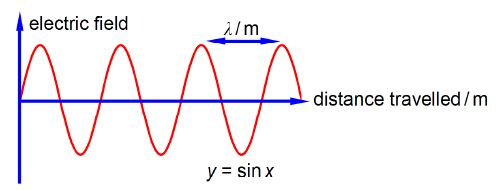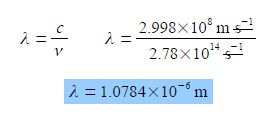# What is Electromagnetic Radiation and Molecular Spectroscopy?

Chemists use spectroscopic analysis all the time in the lab to determine what molecules are present in a sample and what are their concentrations. Spectroscopy is also used as a tool to understand the structure and reactions of molecules. Light, or electromagnetic radiation, consists of a moving wave of electric and magnetic fields which are at right angles to one another and also at right angles to the direction of travel, see below Figure which for clarity only shows the electric field. In order to be able to use spectroscopy in chemistry we must understand light along with the Maths equations which summarize the properties of light.The wavelength λ (Greek letter “lambda”) is the distance between equivalent points on neighbouring waves. The correct symbol for frequency is v (Greek letter “nu”) and it is the number of complete waves which pass a fixed point in a second, the units are “per second” or s-1 (sometimes called hertz). The velocity of light c = 2.998×108 m S-1 is related to its wavelength and its frequency by

c = λ v

If a molecule absorbs light at a frequency of v = 2.78 x 1014 s-1 determine the wavelength A of the light.

Solution

Rearranging the equation so that the unknown quantity λ is the subject of the equation is most clearly done by dividing both sides of the equation by the frequency v.

C = λ v;   c/v = λv / v

Cancelling top and bottom within any single term, then rearranging the equation to make the subject,We say that λ is the subject of the equation when it is on the left of the equal sign and is equal to the rest of the equation. To make this physically meaningful we must substitute the physical quantities, numbers and units, for the symbols and then cancel the units within any single term. In this case it is s-1 that cancels to give the wavelength with the units determined by the correct use of physical quantities.